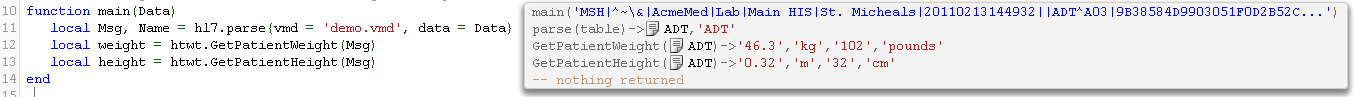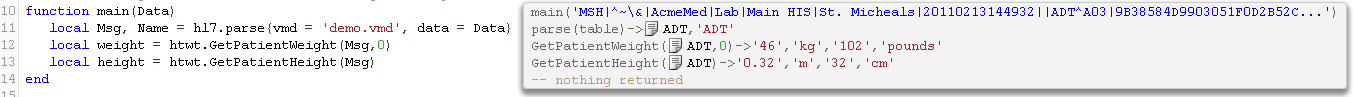# Extracting Height and Weight

This article was originally written for Iguana 5 so it uses version 5 screenshots, and may have some outdated references.

Extracting patient height and weight from an ADT message is more tricky than it sounds. The problem is that this information is typically stored in an OBX segment and encoded as a lab result. For instance:

```MSH|^~\&|AcmeMed|Lab|Main HIS|St. Micheals|20110213144932||ADT^A03|9B38584D9903051F0D2B52CC0148965775D2D23FE4C51BE060B33B6ED27DA820|P|2.6|
EVN||20110213144532||||20110213145902|
NK1|1|Smith^Gary|Second Cousin|
OBX|||WT^WEIGHT||102|pounds|
OBX|||HT^HEIGHT||32|cm|```

One needs to write code to iterate through message, to find the appropriate OBX segment with the right code. Then the units are often non standard. Extracting this data is a problem which lends itself well to writing a reusable module. I have done this and here is an example of using it:You can see that the functions return four values:

1. The quantity converted into metric units.
2. The metric unit.
3. The original value.
4. The original unit.

This makes it easy for the interface programmer to validate that mapping is safe and valid. If weight or height information is not found then the function returns nil. We can optionally supply a second parameter to these functions to determine the rounding. In the next screenshot I tweaked the call for getting the patient weight to round to zero decimal places:It may be necessary to add more units and expected codes that describe height and weight in the module. The code should be relatively clear to read and see where that needs to be done. Be good to get feedback from what variability people see in the field with this information.

## Source code for the htwt patient height and weight extraction library

Here’s the source code for the htwt module. To use it:

1. Create a new shared module called “htwt” and copy paste in the below.
2. Add the code require(‘htwt’) at the top of the main module.
3. Test using sample data.
```htwt={}

function htwt.GetPatientWeight(Msg, Round)
return GetObxProperty(Msg, htwt.Set{"WT", "WEIGHT", "WGT"}, Round)
end

function htwt.GetPatientHeight(Msg, Round)
return GetObxProperty(Msg, htwt.Set{"HT", "HEIGHT", "HGT"}, Round)
end

function GenerateAlias(UnitMap)
for k,v in pairs(UnitMap) do
if not v.alt then break end
for _, Alias in pairs(v.alt) do
UnitMap[Alias] = v
end
end
return UnitMap
end

-- This map assumes we are mapping to metric units.  It is incomplete.  You
-- will need to add more unit definitions.
htwt.unitmap=GenerateAlias{
pound={'kg', 0.45359237, 1, alt={'pounds', 'lb', 'lbs'}},
stone={'kg', 6.35029318, 1, alt={'st'}},
kg   ={'kg', 1,          1, alt={'kilo', 'kilos'}},
cm   ={'m',  .01, 2, alt={'cms', 'centimetre'}},
}

function GetObxProperty(Msg, CodeSet, Round)
local OBX = htwt.findSegment(Msg, htwt.ObxFilter, CodeSet)
if not OBX then return end
local V = OBX:nodeValue()
local U = OBX:nodeValue()
local Vout,Uout = MapUnit(V,U,Round)
end

function htwt.Round(num, idp)
local mult = 10^(idp or 0)
return math.floor(num * mult + 0.5) / mult
end

function MapUnit(V,U, Round)
local M = htwt.unitmap[U:lower()]
if not M then
error("Unit "..U..' is not defined.')
end
U = M
if not Round then Round = M end
local Vout,Uout = MapUnit(V,U,Round)
end

function htwt.Set(List)
local set = {}
for _, V in ipairs(List) do set[V] = true end
return set
end

function htwt.ObxFilter(Segment, CodeSet)
print(CodeSet)
if Segment:nodeName() == 'OBX' then
if CodeSet[Segment]:nodeValue() then
return true
end
end
return false
end

function htwt.findSegment(Msg, Filter, Param)
for i=1, #Msg do
if (Msg[i]:nodeType() == 'segment'
and Filter(Msg[i], Param)) then
return Msg[i]
end
end
for i=1, #Msg do
local T = Msg[i]:nodeType()
if (T == 'segment_group'
or T == 'segment_repeated') then
local R = htwt.findSegment(Msg[i], Filter, Param)
if R ~= nil then
return R
end
end
end
end

function node.S(ANode)